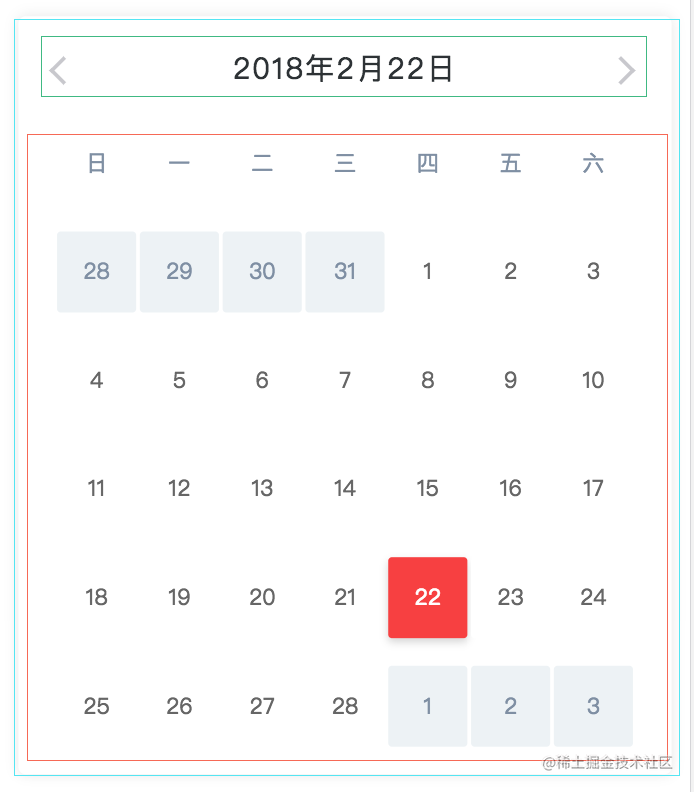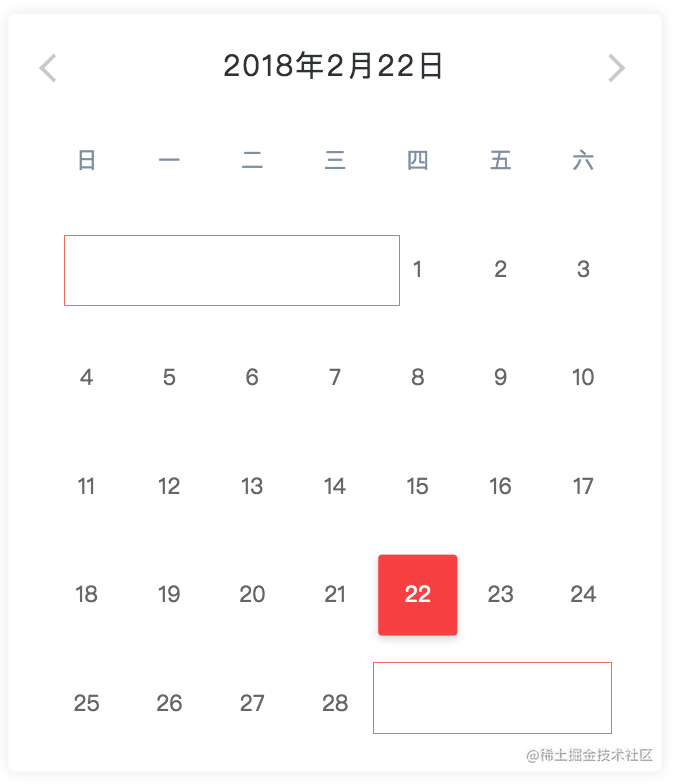# 基于Vue开发一个日历组件

## 更新

• 2.23修复了2026年2月份会渲染多一行的bug，谢谢@深蓝一人童鞋提出的bug，解决方案是给二月份的日历做特殊处理，new Date(year, month+1, 0).getDay() === 6时不会再渲染后面的日期。
• 下班更新一哈，更科学的逻辑
``````// if (total_calendar_list.length > 35) {
//   nextNum = 42 - total_calendar_list.length;
// } else {
//   nextNum = 35 - total_calendar_list.length;
// }

// if (month === 1 && new Date(year, month, 0).getDay() === 6) {
//   nextNum = 0
// }

nextNum = 6 - new Date(year, month+1, 0).getDay()
``````

• 怎么开发一套日历皮肤？
• 怎么计算年月日？
• 怎么开发日历相关的功能？
• 总结&DEMO源码

## 怎么开发一套日历皮肤？

``````// 借鉴了Rem布局
@function pxWithVw(\$n) {
@return 100vw * \$n / 375;
}
// 规定极限宽度，避免PC上观感太差
@function pxWithVwMax(\$n) {
@return 480px * \$n / 375;
}
````````````<!--最外层的div限定整个日历的宽度以及一些圆角阴影等样式-->
<div class="calendar">
<!--顾名思义main则是整个日历的核心内容，也就是日期的展示区域-->
<div class="calendar__main">
<!--星期一~星期日的展示头，列表渲染固定的7个block-->
<!--相应月份的日期展示区域，列表渲染-->
<div class="main__block"></div>
</div>
</div>
``````

## 怎么计算年月日？``````// 定义每个月的天数，如果是闰年第二月改为29天
// year=2018;month=1(js--month=0~11)
let daysInMonth = [31, 28, 31, 30, 31, 30, 31, 31, 30, 31, 30, 31];
if ((year % 4 === 0 && year % 100 !== 0) || year % 400 === 0) {
daysInMonth = 29;
}
// 获得指定年月的1号是星期几
let targetDay = new Date(year, month, 1).getDay();
// 将要在calendar__main中渲染的列表
let total_calendar_list = [];
let preNum = targetDay;
// 首先先说一下，我们的日期是(日--六)这个顺序也就是(0--6)
// 有了上述的前提我们可以认为targetDay为多少，我们就只需要在total_calendar_list的数组中push几个content为''的obj作为占位
if (targetDay > 0) {
for (let i = 0; i < preNum; i++) {
let obj = {
type: "pre",
content: ""
};
total_calendar_list.push(obj);
}
}
``````

``````for (let i = 0; i < daysInMonth[month]; i++) {
let obj = {
type: "normal",
content: i + 1
};
total_calendar_list.push(obj);
}
nextNum = 6 - new Date(year, month+1, 0).getDay()
// 与上面的type=pre同理
for (let i = 0; i < nextNum; i++) {
let obj = {
type: "next",
content: ""
};
total_calendar_list.push(obj);
}
``````

## 怎么开发日历相关的功能？

### 如何选择上一个月或下一个月？

``````data() {
return {
// ...
selectedYear: new Date().getFullYear(),
selectedMonth: new Date().getMonth(),
selectedDate: new Date().getDate()
};
}

handlePreMonth() {
if (this.selectedMonth === 0) {
this.selectedYear = this.selectedYear - 1
this.selectedMonth = 11
this.selectedDate = 1
} else {
this.selectedMonth = this.selectedMonth - 1
this.selectedDate = 1
}
}

handleNextMonth() {
if (this.selectedMonth === 11) {
this.selectedYear = this.selectedYear + 1
this.selectedMonth = 0
this.selectedDate = 1
} else {
this.selectedMonth = this.selectedMonth + 1
this.selectedDate = 1
}
}
``````

### 如果选中某一天？

``````handleDayClick(item) {
if (item.type === 'normal') {
// do anything...
this.selectedDate = Number(item.content)
}
}
``````

## 总结